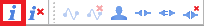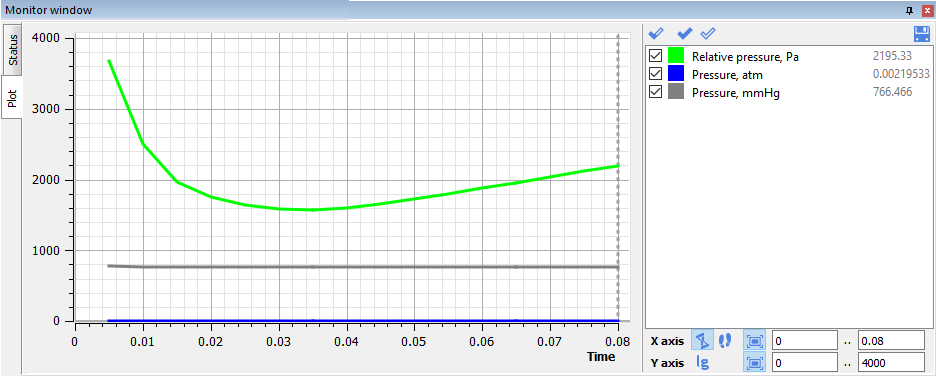### Article Index

Characteristics are one of the most powerful tools in the FlowVision software complex for obtaining information about the calculation.

Characteristics can be used to:

• obtain calculation results, such as: flow rate, pressure, temperature, or forces acting on a surface or in a volume section
• monitor calculation results, outputting the data as a graph within the program interface
• debug the project / diagnose problems (for example, by finding extreme values within the computational space).

### What Are Characteristics

Characteristics are a set of integral values calculated on a selected Object. This can be:

- a standard FlowVision object: plane or box / sphere / cone / cylinder / line / sensor
- an imported object
- a supergroup created on a computational domain boundary or on the surface of a boundary condition
- free space within the volume.

Using Characteristics, you can obtain the value of a variable over a surface or volume of an object, as well as at any point within the domain.

### How to Use Characteristics

You can view the output of created characteristics:

- in the info window at the current time step- in the monitor window as a graph over timeCharacteristic values can be saved to a .glo file for further processing in third-party programs such as Excel, Origin, ParaView.

Characteristics can be calculated:

- within the volume of an object
- over the entire surface of an object
- on individual object surfaces (for example, on the base, side surface, channel surface, or cross-sections)
- along a line (In this case, characteristics are calculated for all cells through which the line passes)
- at a point (In this case, the characteristic tracks the variable values in the cell which contains the point)

Characteristics are useful when calculating secondary variables using the Formula Editor. This approach can be used, for example, to convert values from one numerical system to another.

### Types of Characteristics

##### Internal Characteristics

By default, each project is created with “Internal Characteristics”, which contain information about the main parameters of the calculation: current time, iteration number, time step size, explicit time step value, reference temperature and pressure, gravity vector components. The data from these Characteristics is often necessary to define time or step dependencies in user-defined variables.

##### User Specified Characteristics

Custom characteristics can be created in both the Preprocessor and the Postprocessor.

Due to the sequencing of the FlowVision modular structure, characteristics created in the Postprocessor are not available for use in the Preprocessor

Let’s look at the features of characteristics created on various objects, and consider cases when they can be useful.